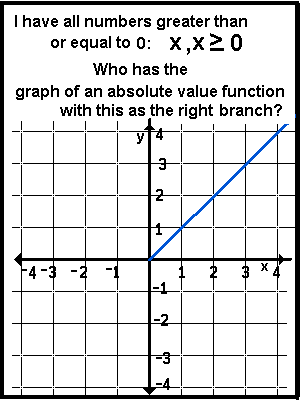### "Who Has" - Function Shifting     © 2004, 2015 A2

For instructions go to: Who Has Intro
This page is provided for the teacher but is not needed to play the game.
Print the copies of the masters and cut them up to make cards.
Print a second copy of the masters to make a transparency
IF THE GAME IS PLAYED ON AN OVERHEAD PROJECTOR.
Master 1  Master 2  Master 3  Master 4  Master 5
Play the game.I have
the graph of the squaring function,
x2.
Who has this graph raised one unit?

I have
the graph of one more than the square of a number,
x2 + 1.
Who has the vertex of this?

I have
(0,1).
Who has the graph of a square root function with
this as the left-most point?

I have
one more than the square root of a number,(x) + 1.
Who has the graph of this moved two places to the right?

I have
one more than the square root of two less than a number,(x - 2) + 1.
Who has the graph of this moved three to the right?

I have
one more than the square root of five less than a number,(x - 5) + 1,
Who has the graph of this moved down one?

I have
the square root of five less than a number,(x - 5),
Who has the graph of the opposite of this?

I have
the opposite of the square root of five less than a number
-(x - 5).
Who has the graph of this moved five to the left?

I have
the opposite of the square root of a number
-(x).
Who has the graph of the opposite of this?

I have
the square root of a number(x).
Who has the square of this?

I have
a number greater than or equal to 0:
x, x > 0.
Who has the graph of an absolute value function with this as the right branch?

I have
the absolute value of a number, the distance a number is from 0,
|x|.
Who has the graph of this moved 2 spaces to the left?

I have the
the distance a number is from - 2
|x + 2|,
Who has the square of this?

I have
the square of two more than a number,
(x + 2)2.
Who has this increased by one?

I have
one more than the square of two more than a number
(x + 2)2 + 1.
Who has the vertex of this?

I have (-2, 1).
Who has this as the intersection of the horizontal & vertical asymptotes of a reciprocal function?

I have
one more than the reciprocal of a number increased by 2.
1/(x+2) + 1.
Who has this moved down one and to the right 2?

I have
the reciprocal of a number ,
1/x.
Who has the opposite of the reciprocal of a number?

I have
the opposite of the reciprocal of a number,
-1/x.
Who has the square of a number?